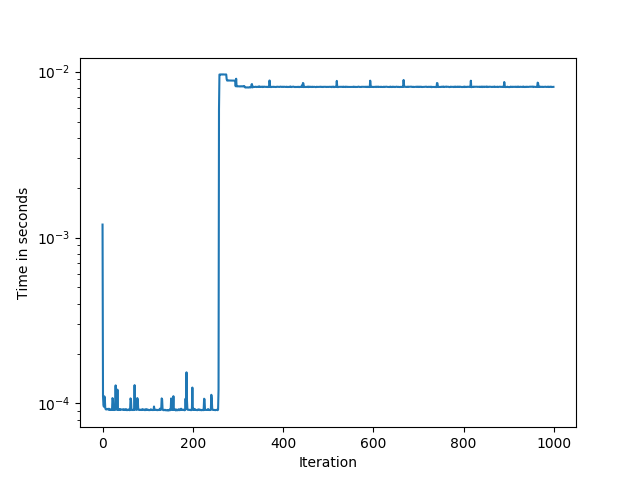# Time increases per iteration of einsum

Hi,

I’m using torch.einsum() in a loop and noting some very strange behaviour, perhaps not related to einsum itself.

When I run it for 10, 100, and 1000 iterations, it takes ~0.003s, ~0.01s, and ~7.3s respectively:

10 trials: 0.0027196407318115234 s
100 trials: 0.010590791702270508 s
1000 trials: 7.267224550247192 s

It seems like the time per call increases drastically after about 250 iterations, see the following plot:To reproduce:

``````import time

import numpy as np
import torch

np.random.seed(123)
device = torch.device('cuda') if torch.cuda.is_available() else torch.device('cpu')

d = 4
p = 500
N = 8000

Z = torch.tensor(np.random.randn(N, p), device=device).float()
V = torch.tensor(np.random.randn(d, p, p), device=device).float()

for trials in [10, 100, 1000]:
start = time.time()
for i in range(trials):
var = torch.einsum("dpq,np,nq->nd", V, Z, Z)
print(f'{trials} trials: {time.time() - start} s')
``````